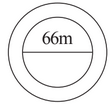Q

# A circular flower bed is surrounded by a path 4 m wide. The diameter of the flower bed is 66 m. What is the area of this path?

Q. 13.     A circular flower bed is surrounded by a path  wide. The diameter of the flower bed is . What is the area of this path?Views

It is given that the diameter of the flower bed is

Therefore,

Now, we know that

Area of the circle (A) =

-(i)

Now, Area of outer circle with radius(r ') = 33 + 4 = 37 cm is

-(ii)

Area of the path is equation (ii) - (i)

Therefore, the area of the path is

Exams
Articles
Questions